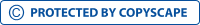Financial ratios, Generally Accepted Accounting Principles, Quick ratioView larger

# Financial ratios, Generally Accepted Accounting Principles, Quick ratio

552313

• High School
• 655

Short excerpt:

A ratio analysis is a tool used by employees to analyze a companyдЫЄs financial statements (Investopedia, 2011). Ratios are calculated from the current year numbers and then compared to another source such as previous years, other companies, or the industry as a whole. This an important tool for evaluating a firmдЫЄs credibility. There are many ratios that are used to determine a ratio analysis. These are short-term solvency ratios, debt management ratios, asset management ratios, profitability ratios, and market value ratios. The two ratios that make up short-term solvency ratios are the current ratio and the quick ratio or acid test ratio. The current ratio uses total current assets and total current liabilities. Total current assets are divided by total current liabilities to give theBy buying this product you can collect up to 26 loyalty points. Your cart will total 26 loyalty points that can be converted into a voucher of \$0.26.

\$2.62